search
Search
Unlock 100+ guides
search toc
close
Cancel
Post
account_circle
Profile
exit_to_app
Sign out
search
keyboard_voice
close
Searching Tips
Search for a recipe:
"Creating a table in MySQL"
Search for an API documentation: "@append"
Search for code: "!dataframe"
Apply a tag filter: "#python"
Useful Shortcuts
/ to open search panel
Esc to close search panel
to navigate between search results
d to clear all current filters
Enter to expand content previewDoc SearchCode Search BetaSORRY NOTHING FOUND!
mic
Start speaking...Voice search is only supported in Safari and Chrome.
Shrink
Navigate to
check_circle
Mark as learned
thumb_up
0
thumb_down
0
chat_bubble_outline
0
Comment
auto_stories Bi-column layout
settings

# Mean Average Precision at K

schedule Aug 12, 2023
Last updated
local_offer
Machine Learning
Tags
expand_more
mode_heat
Master the mathematics behind data science with 100+ top-tier guides
Start your free 7-days trial now!

# Simple example of mean average precision at K

The mean average precision at K, or MAP@K, is a popular evaluation metric for recommendation systems that quantifies how relevant the recommended outputs are to the users.

As as example, suppose we have a total of 100 products and our model recommends 5 products to an user. Suppose for a certain user, the model recommended the following products: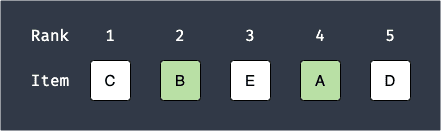Here, note the following:

• the lower the rank, the higher the recommendation score of the product is. This means that product $C$ is a highly recommended product for this particular user.

• the green items are the recommendations that are actually relevant to this user. In this case, products $B$ and $A$ are relevant to the user but products $C$, $E$ and $D$ are not.

Let's start by calculating the quantity called precision@K, where K denotes the number of top K recommendations. The formula for precision@K is as follows:

$$\text{precision@K}= \frac{\text{Number of relevant items in top K recommendations}}{K}$$

For our example, since we are recommending $5$ products to every user, we can calculate precision@K for $k=1,2,3,4,5$ like so: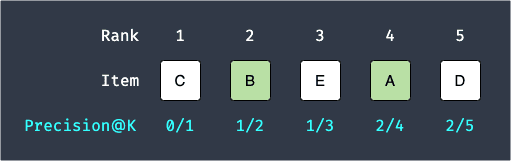Here:

• precision@1 is $0/1$ because the number of relevant items in the top $K=1$ recommendation is $0$.

• precision@2 is $1/2$ because the number of relevant items in the top $K=2$ recommendation is $1$.

To summarize the 5 quantities we have, we compute the so-called average precision@K like so:

$$\begin{equation}\label{eq:Qu9L6xpCwOsOxitlCkF} \text{Average precision@K}= \frac{1}{K} \sum^K_{k=1}I_k\cdot(\text{precision@k}) \end{equation}$$

Where $K$ is the number of recommended products and $I_k$ is the indicator function defined as follows:

$$\begin{equation}\label{eq:zoWQcu0wch47alUx5uk} I_k= \begin{cases} 1,&\text{Item at rank }k\text{ is relevant}\\ 0,&\text{Item at rank }k\text{ is not relevant}\\ \end{cases} \end{equation}$$

For our example, the average precision@5 is:

\begin{align*} \text{Average precision@5}&= \frac{1}{5} \left(0\cdot\frac{0}{1}+1\cdot\frac{1}{2}+ 0\cdot\frac{1}{3}+1\cdot\frac{2}{4}+ 0\cdot\frac{2}{5}\right)\\ &=0.2 \end{align*}

One useful property of average precision@k is that the more relevant the top recommendations are, the higher the value of the average precision@k will be. For instance, consider the following scenario: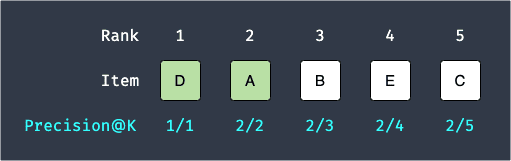The average precision@5 will be:

\begin{align*} \text{Average precision@5}&=\frac{1}{5} \left(1\cdot\frac{1}{1}+1\cdot\frac{2}{2}+ 0\cdot\frac{2}{3}+0\cdot\frac{2}{4}+ 0\cdot\frac{2}{5}\right)\\ &=0.4 \end{align*}

Notice how average precision@5 is larger in this case even though the number of relevant items in the top 5 recommendations are both 2. This is because the average precision@k takes on a higher value if the top recommended products (e.g. rank 1 and 2 items) are relevant. This is useful because we typically want our first few recommendations to be more relevant to the users.

If all the recommended products are relevant, then we achieve the maximum average precision@k of 1. For instance, consider the following case: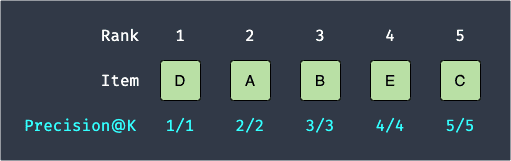In this case, the average precision@5 is:

\begin{align*} \text{Average precision@5}&=\frac{1}{5} \left(1\cdot\frac{1}{1}+1\cdot\frac{2}{2}+ 1\cdot\frac{3}{3}+1\cdot\frac{4}{4}+ 1\cdot\frac{5}{5}\right)\\ &=1 \end{align*}

What we have discussed up to now applies to recommendations made to a single user. If our model makes recommendations to $n$ number of users, then we should compute the mean of the average precision@k across the $n$ users. This gives us the so-called mean average precision@k, whose formula is given below:

\begin{align*} \text{Mean average precision@k}&= \frac{1}{n}\sum^n_{i=1}\left(\frac{1}{K} \sum^K_{k=1}I_{k,i}\cdot(\text{precision@k})_i\right) \end{align*}

Where:

• $n$ is the number of users.

• $K$ is the number of recommendations.

• $I_{k,i}$ is the indicator function \eqref{eq:zoWQcu0wch47alUx5uk} for the $i$-th user.

• $(\mathrm{precision@k})_i$ is the $\text{precision@k}$ value for the $i$-th user.

As an example, suppose we have 2 users and we make the following 5 recommendations to each user: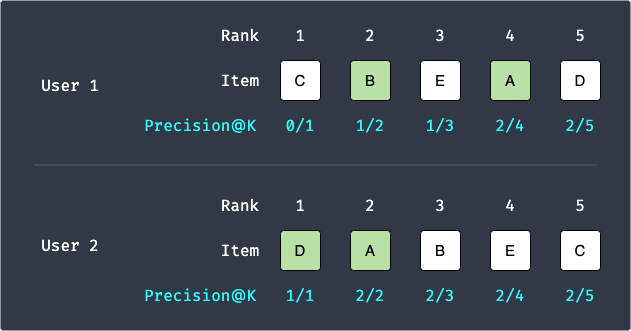We've already computed the average precision@5 for both cases earlier:

\begin{align*} \text{User 1: Average precision@5}&= \frac{1}{5} \left(0\cdot\frac{0}{1}+1\cdot\frac{1}{2}+ 0\cdot\frac{1}{3}+1\cdot\frac{2}{4}+ 0\cdot\frac{2}{5}\right)=0.2\\ \text{User 2: Average precision@5}&=\frac{1}{5} \left(1\cdot\frac{1}{1}+1\cdot\frac{2}{2}+ 0\cdot\frac{2}{3}+0\cdot\frac{2}{4}+ 0\cdot\frac{2}{5}\right)=0.4 \end{align*}

Since $n=2$, the mean average precision@5 is:

\begin{align*} \text{Mean average precision@5}&= \frac{1}{2}(0.2+0.4)\\ &=0.3 \end{align*}

Just like average precision@K, the highest value that the mean average precision@K can take is $1$.

# Variant of mean average precision at K

Recall from \eqref{eq:Qu9L6xpCwOsOxitlCkF} that the average precision@K is calculated like so:

$$\text{Average precision@K}= \frac{1}{K} \sum^K_{k=1}I_k\cdot(\text{precision@k})$$

It is possible to achieve the maximum average precision@K value of $1$ if all the recommendations are relevant - we demonstrated this earlier. However, if the number of relevant items that the user has is less than K, then the average precision@K drops - no matter what recommendations the model outputs for this user, some of them would not be relevant.

For example, suppose a user only likes items B and E but no other items. If our model provides 5 recommendations, then no matter how great the model is, there will always be at least 3 irrelevant items within the 5 recommended items. As a result, the average precision@K drops. This is unfair for the model because it is bound to recommend irrelevant items for this user. To account for this, we sometimes use the following variant instead:

$$\begin{equation}\label{eq:mPKip1gJr7AMwVB3vbZ} \text{Average precision@K}= \frac{1}{\min(r,K)} \sum^K_{k=1}I_k\cdot(\text{precision@k}) \end{equation}$$

Where $r$ is number of products that the user finds relevant out of all the products.

Let's now go through an example. Out of all $1000$ products, suppose a user only finds $3$ items (e.g. items A, B and F) that are relevant, that is, $r=3$. Again, suppose our model provides $5$ recommendations to this user, which means $K=5$. Finally, let's assume that our model outputs the following 5 recommendations: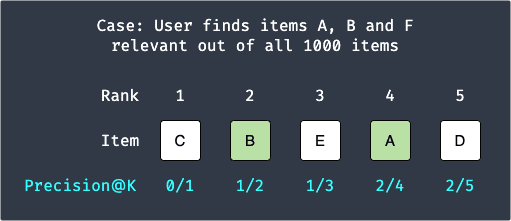Applying formula \eqref{eq:mPKip1gJr7AMwVB3vbZ} gives:

\begin{align*} \text{Average precision@5}&= \frac{1}{\min(3,5)} \left(0\cdot\frac{0}{1}+1\cdot\frac{1}{2}+ 0\cdot\frac{1}{3}+1\cdot\frac{2}{4}+ 0\cdot\frac{2}{5}\right)\\ &\approx0.33 \end{align*}

The reason why we use a $\text{min}$ operator is to ensure that the metric is not penalized for having excess number of relevant items. For instance, consider the extreme case when a user finds all $r=1000$ items relevant. Without the $\text{min}$ operator, the average precision@5 is:

\begin{align*} \text{Average precision@5}&= \frac{1}{1000} \left( 1\cdot\frac{1}{1}+1\cdot\frac{2}{2}+ 1\cdot\frac{3}{3}+1\cdot\frac{4}{4}+ 1\cdot\frac{5}{5}\right)\\ &\approx0.005 \end{align*}

This is misleading because even though it managed to output all relevant items to the users, it received an extremely low score of $0.005$. Therefore, we cap the denominator using a $\text{min}$ operator like so:

\begin{align*} \text{Average precision@5}&= \frac{1}{\text{min}(5,1000)} \left( 1\cdot\frac{1}{1}+1\cdot\frac{2}{2}+ 1\cdot\frac{3}{3}+1\cdot\frac{4}{4}+ 1\cdot\frac{5}{5}\right)\\ &=1 \end{align*}

This is more accurate because our recommendations system has done the perfect job of recommending relevant items to the users.

WARNING

Whether the $\min$ operator is used actually depends on the implementation. For example, PySpark's implementationopen_in_new of MAP@K does not - instead of $K$, we use $r$ for the denominator:

$$\text{Average precision@K}= \frac{1}{r} \sum^K_{k=1}I_k\cdot(\text{precision@k})$$

# Python implementation of MAP@K

Before implementing MAP@K, let's first implement AP@K. To motivate our implementation, let's revisit the example from earlier:We've already calculated the AP@5 for this particular set of recommendations:

\begin{align*} \text{Average precision@5}&= \frac{1}{\min(3,5)} \left(0\cdot\frac{0}{1}+1\cdot\frac{1}{2}+ 0\cdot\frac{1}{3}+1\cdot\frac{2}{4}+ 0\cdot\frac{2}{5}\right)\\ &\approx0.33 \end{align*}

The Python implementation for AP@K using the library numpy is:

 import numpy as npdef apk(recs, rels): x = 0 sum = 0 for i, rec_id in enumerate(recs): is_rel = (rec_id in rels) mult = 1 if is_rel else 0 if is_rel: x += 1 sum += mult * (x / (i+1)) return sum / min(len(rels), len(recs))recs = ["C","B","E","A","D"]rels = ["A","B","F"]apk(recs, rels) 0.3333333333333333 

Now, recall that MAP@K is simply the average of average precision@K across all users. Therefore, the implementation of MAP@K is:

 all_recs = [["C","B","E","A","D"], ["C","E","A","F","B"]]all_rels = [["A","B","F"], "F"]def mapk(all_recs, all_rels): sum = 0 for recs, rels in zip(all_recs, all_rels): sum += apk(recs, rels) return sum / len(all_recs)mapk(all_recs, all_rels) 0.29166666666666663 
thumb_up
thumb_down
Comment
Citation
Ask a question or leave a feedback...
thumb_up
0
thumb_down
0
chat_bubble_outline
0
settings
Enjoy our search
Hit / to insta-search docs and recipes!